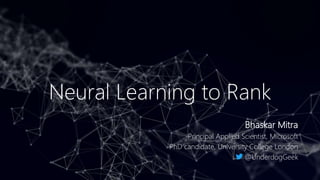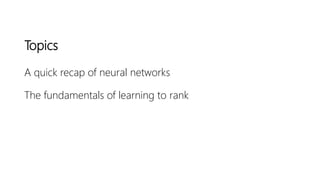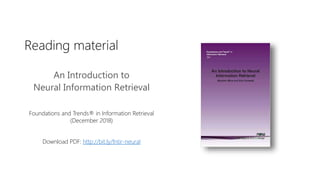Successfully reported this slideshow.

# Neural Learning to Rank×

1 of 47
1 of 47

# Neural Learning to Rank

Learning to rank (LTR) for information retrieval (IR) involves the application of machine learning models to rank artifacts, such as items to be recommended, in response to user's need. LTR models typically employ training data, such as human relevance labels and click data, to discriminatively train towards an IR objective. The focus of this tutorial will be on the fundamentals of neural networks and their applications to learning to rank.

Learning to rank (LTR) for information retrieval (IR) involves the application of machine learning models to rank artifacts, such as items to be recommended, in response to user's need. LTR models typically employ training data, such as human relevance labels and click data, to discriminatively train towards an IR objective. The focus of this tutorial will be on the fundamentals of neural networks and their applications to learning to rank.

### Neural Learning to Rank

1. 1. Neural Learning to Rank Bhaskar Mitra Principal Applied Scientist, Microsoft PhD candidate, University College London @UnderdogGeek
2. 2. Topics A quick recap of neural networks The fundamentals of learning to rank
3. 3. Reading material An Introduction to Neural Information Retrieval Foundations and Trends® in Information Retrieval (December 2018) Download PDF: http://bit.ly/fntir-neural
4. 4. Most information retrieval (IR) systems present a ranked list of retrieved artifacts
5. 5. Learning to Rank (LTR) ”... the task to automatically construct a ranking model using training data, such that the model can sort new objects according to their degrees of relevance, preference, or importance.” - Liu  Tie-Yan Liu. Learning to rank for information retrieval. Foundation and Trends in Information Retrieval, 2009. Image source: https://storage.googleapis.com/pub-tools-public-publication-data/pdf/45530.pdf
6. 6. A quick recap of neural networks
7. 7. Vectors, matrices, and tensors Image source: https://dev.to/mmithrakumar/scalars-vectors-matrices-and-tensors-with-tensorflow-2-0-1f66 Image source: https://hadrienj.github.io/posts/Deep-Learning-Book-Series-2.1-Scalars-Vectors-Matrices-and-Tensors/ matrix transpose matrix addition dot product matrix multiplication
9. 9. Neural networks Chains of parameterized linear transforms (e.g., multiply weight, add bias) followed by non-linear functions (σ) Popular choices for σ: Parameters trained using backpropagation E2E training over millions of samples in batched mode Many choices of architecture and hyper-parameters Non-linearity Input Linear transform Non-linearity Linear transform Predicted output forwardpass backwardpass Expected output loss Tanh ReLU
10. 10. Basic machine learning tasks
11. 11. Squared loss The squared loss is a popular loss function for regression tasks
12. 12. The softmax function In neural classification models, the softmax function is popularly used to normalize the neural network output scores across all the classes
13. 13. Cross entropy The cross entropy between two probability distributions 𝑝 and 𝑞 over a discrete set of events is given by, If 𝑝 𝑐𝑜𝑟𝑟𝑒𝑐𝑡 = 1and 𝑝𝑖 = 0 for all other values of 𝑖 then,
14. 14. Cross entropy with softmax loss Cross entropy with softmax is a popular loss function for classification
15. 15. We are given training data: < 𝑥, 𝑦 > pairs, where 𝑥 is input and 𝑦 is expected output Step 1: Define model and randomly initialize learnable model parameters Step 2: Given 𝑥, compute model output Step 3: Given model output and 𝑦, compute loss 𝑙 Step 4: Compute gradient 𝜕𝑙 𝜕𝑤 of loss 𝑙 w.r.t. each parameter 𝑤 Step 5: Update parameter as 𝑤 𝑛𝑒𝑤 = 𝑤 𝑜𝑙𝑑 − 𝜂 × 𝜕𝑙 𝜕𝑤 , where 𝜂 is learning rate Step 6: Go back to step 2 and repeat till convergence Gradient Descent
16. 16. Goal: iteratively update the learnable parameters such that the loss 𝑙 is minimized Compute the gradient of the loss 𝑙 w.r.t. each parameter (e.g., 𝑤1) 𝜕𝑙 𝜕𝑤1 = 𝜕𝑙 𝜕𝑦2 × 𝜕𝑦2 𝜕𝑦1 × 𝜕𝑦1 𝜕𝑤1 Update the parameter value based on the gradient with 𝜂 as the learning rate 𝑤1 𝑛𝑒𝑤 = 𝑤1 𝑜𝑙𝑑 − 𝜂 × 𝜕𝑙 𝜕𝑤1 Gradient Descent Task: regression Training data: 𝑥, 𝑦 pairs Model: NN (1 feature, 1 hidden layer, 1 hidden node) Learnable parameters: 𝑤1, 𝑏1, 𝑤2, 𝑏2 𝑥 𝑦1 𝑦2 𝑙 𝑡𝑎𝑛ℎ 𝑤1. 𝑥 + 𝑏1 𝑦 − 𝑦2 2 𝑦 𝑡𝑎𝑛ℎ 𝑤2. 𝑦1 + 𝑏2 …and repeat
17. 17. Goal: iteratively update the learnable parameters such that the loss 𝑙 is minimized Compute the gradient of the loss 𝑙 w.r.t. each parameter (e.g., 𝑤1) 𝜕𝑙 𝜕𝑤1 = 𝜕 𝑦 − 𝑦2 2 𝜕𝑦2 × 𝜕𝑦2 𝜕𝑦1 × 𝜕𝑦1 𝜕𝑤1 Update the parameter value based on the gradient with 𝜂 as the learning rate 𝑤1 𝑛𝑒𝑤 = 𝑤1 𝑜𝑙𝑑 − 𝜂 × 𝜕𝑙 𝜕𝑤1 Gradient Descent Task: regression Training data: 𝑥, 𝑦 pairs Model: NN (1 feature, 1 hidden layer, 1 hidden node) Learnable parameters: 𝑤1, 𝑏1, 𝑤2, 𝑏2 𝑥 𝑦1 𝑦2 𝑙 𝑡𝑎𝑛ℎ 𝑤1. 𝑥 + 𝑏1 𝑦 − 𝑦2 2 𝑦 𝑡𝑎𝑛ℎ 𝑤2. 𝑦1 + 𝑏2 …and repeat
18. 18. Goal: iteratively update the learnable parameters such that the loss 𝑙 is minimized Compute the gradient of the loss 𝑙 w.r.t. each parameter (e.g., 𝑤1) 𝜕𝑙 𝜕𝑤1 = −2 × 𝑦 − 𝑦2 × 𝜕𝑦2 𝜕𝑦1 × 𝜕𝑦1 𝜕𝑤1 Update the parameter value based on the gradient with 𝜂 as the learning rate 𝑤1 𝑛𝑒𝑤 = 𝑤1 𝑜𝑙𝑑 − 𝜂 × 𝜕𝑙 𝜕𝑤1 Gradient Descent Task: regression Training data: 𝑥, 𝑦 pairs Model: NN (1 feature, 1 hidden layer, 1 hidden node) Learnable parameters: 𝑤1, 𝑏1, 𝑤2, 𝑏2 𝑥 𝑦1 𝑦2 𝑙 𝑡𝑎𝑛ℎ 𝑤1. 𝑥 + 𝑏1 𝑦 − 𝑦2 2 𝑦 𝑡𝑎𝑛ℎ 𝑤2. 𝑦1 + 𝑏2 …and repeat
19. 19. Goal: iteratively update the learnable parameters such that the loss 𝑙 is minimized Compute the gradient of the loss 𝑙 w.r.t. each parameter (e.g., 𝑤1) 𝜕𝑙 𝜕𝑤1 = −2 × 𝑦 − 𝑦2 × 𝜕𝑡𝑎𝑛ℎ 𝑤2. 𝑦1 + 𝑏2 𝜕𝑦1 × 𝜕𝑦1 𝜕𝑤1 Update the parameter value based on the gradient with 𝜂 as the learning rate 𝑤1 𝑛𝑒𝑤 = 𝑤1 𝑜𝑙𝑑 − 𝜂 × 𝜕𝑙 𝜕𝑤1 Gradient Descent Task: regression Training data: 𝑥, 𝑦 pairs Model: NN (1 feature, 1 hidden layer, 1 hidden node) Learnable parameters: 𝑤1, 𝑏1, 𝑤2, 𝑏2 𝑥 𝑦1 𝑦2 𝑙 𝑡𝑎𝑛ℎ 𝑤1. 𝑥 + 𝑏1 𝑦 − 𝑦2 2 𝑦 𝑡𝑎𝑛ℎ 𝑤2. 𝑦1 + 𝑏2 …and repeat
20. 20. Goal: iteratively update the learnable parameters such that the loss 𝑙 is minimized Compute the gradient of the loss 𝑙 w.r.t. each parameter (e.g., 𝑤1) 𝜕𝑙 𝜕𝑤1 = −2 × 𝑦 − 𝑦2 × 1 − 𝑡𝑎𝑛ℎ2 𝑤2. 𝑥 + 𝑏2 × 𝑤2 × 𝜕𝑦1 𝜕𝑤1 Update the parameter value based on the gradient with 𝜂 as the learning rate 𝑤1 𝑛𝑒𝑤 = 𝑤1 𝑜𝑙𝑑 − 𝜂 × 𝜕𝑙 𝜕𝑤1 Gradient Descent Task: regression Training data: 𝑥, 𝑦 pairs Model: NN (1 feature, 1 hidden layer, 1 hidden node) Learnable parameters: 𝑤1, 𝑏1, 𝑤2, 𝑏2 𝑥 𝑦1 𝑦2 𝑙 𝑡𝑎𝑛ℎ 𝑤1. 𝑥 + 𝑏1 𝑦 − 𝑦2 2 𝑦 𝑡𝑎𝑛ℎ 𝑤2. 𝑦1 + 𝑏2 …and repeat
21. 21. Goal: iteratively update the learnable parameters such that the loss 𝑙 is minimized Compute the gradient of the loss 𝑙 w.r.t. each parameter (e.g., 𝑤1) 𝜕𝑙 𝜕𝑤1 = −2 × 𝑦 − 𝑦2 × 1 − 𝑡𝑎𝑛ℎ2 𝑤2. 𝑥 + 𝑏2 × 𝑤2 × 𝜕𝑡𝑎𝑛ℎ 𝑤1. 𝑥 + 𝑏1 𝜕𝑤1 Update the parameter value based on the gradient with 𝜂 as the learning rate 𝑤1 𝑛𝑒𝑤 = 𝑤1 𝑜𝑙𝑑 − 𝜂 × 𝜕𝑙 𝜕𝑤1 Gradient Descent Task: regression Training data: 𝑥, 𝑦 pairs Model: NN (1 feature, 1 hidden layer, 1 hidden node) Learnable parameters: 𝑤1, 𝑏1, 𝑤2, 𝑏2 𝑥 𝑦1 𝑦2 𝑙 𝑡𝑎𝑛ℎ 𝑤1. 𝑥 + 𝑏1 𝑦 − 𝑦2 2 𝑦 𝑡𝑎𝑛ℎ 𝑤2. 𝑦1 + 𝑏2 …and repeat
22. 22. Goal: iteratively update the learnable parameters such that the loss 𝑙 is minimized Compute the gradient of the loss 𝑙 w.r.t. each parameter (e.g., 𝑤1) 𝜕𝑙 𝜕𝑤1 = −2 × 𝑦 − 𝑦2 × 1 − 𝑡𝑎𝑛ℎ2 𝑤2. 𝑥 + 𝑏2 × 𝑤2 × 1 − 𝑡𝑎𝑛ℎ2 𝑤1. 𝑥 + 𝑏1 × 𝑥 Update the parameter value based on the gradient with 𝜂 as the learning rate 𝑤1 𝑛𝑒𝑤 = 𝑤1 𝑜𝑙𝑑 − 𝜂 × 𝜕𝑙 𝜕𝑤1 Gradient Descent Task: regression Training data: 𝑥, 𝑦 pairs Model: NN (1 feature, 1 hidden layer, 1 hidden node) Learnable parameters: 𝑤1, 𝑏1, 𝑤2, 𝑏2 𝑥 𝑦1 𝑦2 𝑙 𝑡𝑎𝑛ℎ 𝑤1. 𝑥 + 𝑏1 𝑦 − 𝑦2 2 𝑦 𝑡𝑎𝑛ℎ 𝑤2. 𝑦1 + 𝑏2 …and repeat
23. 23. Exercise Simple Neural Network from Scratch Implement a simple multi-layer neural network with single input feature, single output, and single neuron per layer using (i) PyTorch and (ii) from scratch—and demonstrate that both approaches produce identical outcome. https://github.com/spacemanidol/AFIRMDeep Learning2020/blob/master/NNPrimer.ipynb
24. 24. Computation Networks The “Lego” approach to specifying neural architectures Library of neural layers, each layer defines logic for: 1. Forward pass: compute layer output given layer input 2. Backward pass: a) compute gradient of layer output w.r.t. layer inputs b) compute gradient of layer output w.r.t. layer parameters (if any) Chain nodes to create bigger and more complex networks
25. 25. Why adding depth helps http://playground.tensorflow.org
27. 27. Bias-variance trade-off in the deep learning era Mikhail Belkin, Daniel Hsu, Siyuan Ma, and Soumik Mandal. Reconciling modern machine-learning practice and the classical bias–variance trade-off. In PNAS, 2019.
28. 28. Jonathan Frankle and Michael Carbin. The lottery ticket hypothesis: Finding sparse, trainable neural networks. In ICLR, 2019. Vivek Ramanujan, Mitchell Wortsman, Aniruddha Kembhavi, Ali Farhadi, and Mohammad Rastegari. What's Hidden in a Randomly Weighted Neural Network? In ArXiv, 2019. The lottery ticket hypothesis
29. 29. Questions?
30. 30. The fundamentals of learning to rank
31. 31. Problem formulation LTR models represent a rankable item—e.g., a document or a movie or a song—given some context—e.g., a user-issued query or user’s historical interactions with other items—as a numerical vector 𝑥 ∈ ℝ 𝑛 The ranking model 𝑓: 𝑥 → ℝ is trained to map the vector to a real-valued score such that relevant items are scored higher.
32. 32. Why is ranking challenging? Examples of ranking metrics Discounted Cumulative Gain (DCG) 𝐷𝐶𝐺@𝑘 = 𝑖=1 𝑘 2 𝑟𝑒𝑙𝑖 − 1 𝑙𝑜𝑔2 𝑖 + 1 Reciprocal Rank (RR) 𝑅𝑅@𝑘 = max 1<𝑖<𝑘 𝑟𝑒𝑙𝑖 𝑖 Rank based metrics, such as DCG and MRR, are non-smooth / non-differentiable
33. 33. Features They can often be categorized as: Query-independent or static features e.g., incoming link count and document length Query-dependent or dynamic features e.g., BM25 Query-level features e.g., query length Traditional L2R models employ hand-crafted features that encode IR insights
34. 34. Features Tao Qin, Tie-Yan Liu, Jun Xu, and Hang Li. LETOR: A Benchmark Collection for Research on Learning to Rank for Information Retrieval, Information Retrieval Journal, 2010
35. 35. Approaches Pointwise approach Relevance label 𝑦 𝑞,𝑑 is a number—derived from binary or graded human judgments or implicit user feedback (e.g., CTR). Typically, a regression or classification model is trained to predict 𝑦 𝑞,𝑑 given 𝑥 𝑞,𝑑. Pairwise approach Pairwise preference between documents for a query (𝑑𝑖 ≻ 𝑑𝑗 w.r.t. 𝑞) as label. Reduces to binary classification to predict more relevant document. Listwise approach Directly optimize for rank-based metric, such as NDCG—difficult because these metrics are often not differentiable w.r.t. model parameters. Liu  categorizes different LTR approaches based on training objectives: Tie-Yan Liu. Learning to rank for information retrieval. Foundation and Trends in Information Retrieval, 2009.
36. 36. Pointwise objectives Regression loss Given 𝑞, 𝑑 predict the value of 𝑦 𝑞,𝑑 e.g., square loss for binary or categorical labels, where, 𝑦 𝑞,𝑑 is the one-hot representation [Fuhr, 1989] or the actual value [Cossock and Zhang, 2006] of the label Norbert Fuhr. Optimum polynomial retrieval functions based on the probability ranking principle. ACM TOIS, 1989. David Cossock and Tong Zhang. Subset ranking using regression. In COLT, 2006. labels prediction 0 1 1
37. 37. Pointwise objectives Classification loss Given 𝑞, 𝑑 predict the class 𝑦 𝑞,𝑑 e.g., cross-entropy with softmax over categorical labels 𝑌 [Li et al., 2008], where, 𝑠 𝑦 𝑞,𝑑 is the model’s score for label 𝑦 𝑞,𝑑 labels prediction 0 1 Ping Li, Qiang Wu, and Christopher J Burges. Mcrank: Learning to rank using multiple classification and gradient boosting. In NIPS, 2008.
38. 38. Pairwise objectives Pairwise loss generally has the following form [Chen et al., 2009], where, 𝜙 can be, • Hinge function 𝜙 𝑧 = 𝑚𝑎𝑥 0, 1 − 𝑧 [Herbrich et al., 2000] • Exponential function 𝜙 𝑧 = 𝑒−𝑧 [Freund et al., 2003] • Logistic function 𝜙 𝑧 = 𝑙𝑜𝑔 1 + 𝑒−𝑧 [Burges et al., 2005] • Others… Pairwise loss minimizes the average number of inversions in ranking—i.e., 𝑑𝑖 ≻ 𝑑𝑗 w.r.t. 𝑞 but 𝑑𝑗 is ranked higher than 𝑑𝑖 Given 𝑞, 𝑑𝑖, 𝑑𝑗 , predict the more relevant document For 𝑞, 𝑑𝑖 and 𝑞, 𝑑𝑗 , Feature vectors: 𝑥𝑖 and 𝑥𝑗 Model scores: 𝑠𝑖 = 𝑓 𝑥𝑖 and 𝑠𝑗 = 𝑓 𝑥𝑗 Wei Chen, Tie-Yan Liu, Yanyan Lan, Zhi-Ming Ma, and Hang Li. Ranking measures and loss functions in learning to rank. In NIPS, 2009. Ralf Herbrich, Thore Graepel, and Klaus Obermayer. Large margin rank boundaries for ordinal regression. 2000. Yoav Freund, Raj Iyer, Robert E Schapire, and Yoram Singer. An efficient boosting algorithm for combining preferences. In JMLR, 2003. Chris Burges, Tal Shaked, Erin Renshaw, Ari Lazier, Matt Deeds, Nicole Hamilton, and Greg Hullender. Learning to rank using gradient descent. In ICML, 2005.
39. 39. Pairwise objectives RankNet loss Pairwise loss function proposed by Burges et al. —an industry favourite [Burges, 2015] Predicted probabilities: 𝑝𝑖𝑗 = 𝑝 𝑠𝑖 > 𝑠𝑗 ≡ 𝑒 𝛾.𝑠 𝑖 𝑒 𝛾.𝑠 𝑖 +𝑒 𝛾.𝑠 𝑗 = 1 1+𝑒 −𝛾. 𝑠 𝑖−𝑠 𝑗 Desired probabilities: 𝑝𝑖𝑗 = 1 and 𝑝𝑗𝑖 = 0 Computing cross-entropy between 𝑝 and 𝑝 ℒ 𝑅𝑎𝑛𝑘𝑁𝑒𝑡 = − 𝑝𝑖𝑗. 𝑙𝑜𝑔 𝑝𝑖𝑗 − 𝑝𝑗𝑖. 𝑙𝑜𝑔 𝑝𝑗𝑖 = −𝑙𝑜𝑔 𝑝𝑖𝑗 = 𝑙𝑜𝑔 1 + 𝑒−𝛾. 𝑠 𝑖−𝑠 𝑗 pairwise preference score 0 1 Chris Burges, Tal Shaked, Erin Renshaw, Ari Lazier, Matt Deeds, Nicole Hamilton, and Greg Hullender. Learning to rank using gradient descent. In ICML, 2005. Chris Burges. RankNet: A ranking retrospective. https://www.microsoft.com/en-us/research/blog/ranknet-a-ranking-retrospective/. 2015.
40. 40. A generalized cross-entropy loss An alternative loss function assumes a single relevant document 𝑑+ and compares it against the full collection 𝐷 Predicted probabilities: p 𝑑+|𝑞 = 𝑒 𝛾.𝑠 𝑞,𝑑+ 𝑑∈𝐷 𝑒 𝛾.𝑠 𝑞,𝑑 The cross-entropy loss is then given by, ℒ 𝐶𝐸 𝑞, 𝑑+, 𝐷 = −𝑙𝑜𝑔 p 𝑑+|𝑞 = −𝑙𝑜𝑔 𝑒 𝛾.𝑠 𝑞,𝑑+ 𝑑∈𝐷 𝑒 𝛾.𝑠 𝑞,𝑑 Computing the softmax over the full collection is prohibitively expensive—LTR models typically consider few negative candidates [Huang et al., 2013, Shen et al., 2014, Mitra et al., 2017] Po-Sen Huang, Xiaodong He, Jianfeng Gao, Li Deng, Alex Acero, and Larry Heck. Learning deep structured semantic models for web search using clickthrough data. In CIKM, 2013. Yelong Shen, Xiaodong He, Jianfeng Gao, Li Deng, and Gregoire Mesnil. A latent semantic model with convolutional-pooling structure for information retrieval. In CIKM, 2014. Bhaskar Mitra, Fernando Diaz, and Nick Craswell. Learning to match using local and distributed representations of text for web search. In WWW, 2017.
41. 41. Blue: relevant Gray: non-relevant NDCG and ERR higher for left but pairwise errors less for right Due to strong position-based discounting in IR measures, errors at higher ranks are much more problematic than at lower ranks But listwise metrics are non-continuous and non-differentiable LISTWISE OBJECTIVES Christopher JC Burges. From ranknet to lambdarank to lambdamart: An overview. Learning, 2010. [Burges, 2010]
42. 42. Listwise objectives Burges et al.  make two observations: 1. To train a model we don’t need the costs themselves, only the gradients (of the costs w.r.t model scores) 2. It is desired that the gradient be bigger for pairs of documents that produces a bigger impact in NDCG by swapping positions Christopher JC Burges, Robert Ragno, and Quoc Viet Le. Learning to rank with nonsmooth cost functions. In NIPS, 2006. LambdaRank loss Multiply actual gradients with the change in NDCG by swapping the rank positions of the two documents
43. 43. Listwise objectives According to the Luce model [Luce, 2005], given four items 𝑑1, 𝑑2, 𝑑3, 𝑑4 the probability of observing a particular rank-order, say 𝑑2, 𝑑1, 𝑑4, 𝑑3 , is given by: where, 𝜋 is a particular permutation and 𝜙 is a transformation (e.g., linear, exponential, or sigmoid) over the score 𝑠𝑖 corresponding to item 𝑑𝑖 R Duncan Luce. Individual choice behavior. 1959. Zhe Cao, Tao Qin, Tie-Yan Liu, Ming-Feng Tsai, and Hang Li. Learning to rank: from pairwise approach to listwise approach. In ICML, 2007. Fen Xia, Tie-Yan Liu, Jue Wang, Wensheng Zhang, and Hang Li. Listwise approach to learning to rank: theory and algorithm. In ICML, 2008. ListNet loss Cao et al.  propose to compute the probability distribution over all possible permutations based on model score and ground- truth labels. The loss is then given by the K-L divergence between these two distributions. This is computationally very costly, computing permutations of only the top-K items makes it slightly less prohibitive. ListMLE loss Xia et al.  propose to compute the probability of the ideal permutation based on the ground truth. However, with categorical labels more than one permutation is possible.
44. 44. Listwise objectives Mingrui Wu, Yi Chang, Zhaohui Zheng, and Hongyuan Zha. Smoothing DCG for learning to rank: A novel approach using smoothed hinge functions. In CIKM, 2009. Smooth DCG Wu et al.  compute a “smooth” rank of documents as a function of their scores This “smooth” rank can be plugged into a ranking metric, such as MRR or DCG, to produce a smooth ranking loss
45. 45. Questions? @UnderdogGeek bmitra@microsoft.com